# Perspective

(diff) ← Older revision | Latest revision (diff) | Newer revision → (diff)
Jump to: navigation, search

with centreA mapping of a planeinto a plane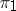under which each pointinis put into correspondence with the point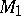of intersection of the straight line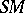with(ifis not parallel to, see Fig.).Figure: p072410a

More generally, letand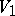be proper subspaces of identical dimension in a projective spaceand letbe a subspace of maximal dimension not having points in common withor. Letbe a subspace contained in, letbe the subspace of minimal dimension containingandand let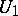be the intersection ofand.

The correspondence by which each subspacecontained inis put into correspondence with the subspacecontained inis called a perspective mapping fromintowith perspective centre.

A perspective is a collineation. If the subspacesandintersect, then each point in the subspace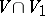corresponds to itself.

If projective coordinates are introduced into the spacesand, then a perspective correspondence can be specified by a linear mapping.

How to Cite This Entry:
Perspective. Encyclopedia of Mathematics. URL: http://encyclopediaofmath.org/index.php?title=Perspective&oldid=18072
This article was adapted from an original article by P.S. Modenov (originator), which appeared in Encyclopedia of Mathematics - ISBN 1402006098. See original article